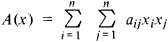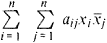Also found in: Wikipedia.

(mathematics)
Any second-degree, homogeneous polynomial.
McGraw-Hill Dictionary of Scientific & Technical Terms, 6E, Copyright © 2003 by The McGraw-Hill Companies, Inc.
The following article is from The Great Soviet Encyclopedia (1979). It might be outdated or ideologically biased.

a second-degree form in n variables x1, x2. …, xn that is, a polynomial of these variables, each term of which contains either the square of one of the variables or the product of two different variables. The general form of a quadratic form for n = 2 is

ax12 + bx22 + cx1x2

and for n = 3,

ax12 + bx22 + bc32 + dx1x2 + ex1x3 + fx2x3

where a, b, …, fare any number. An arbitrary quadratic form is written aswhere it is assumed that aij = aji. Quadratic forms in two, three, and four variables are directly connected with the theory of second-order curves (in the plane) and surfaces (in space): in Cartesian coordinates the equation of a centrally symmetric second-order curve or surface has the form A(x) = 1, where A (x) is a quadratic form. In homogeneous coordinates the left-hand side of any equation of a second-order curve or surface is a quadratic form. Upon repacement of the variables x1, x2, …, xn by other variables y1, y2yn, which are linear combinations of the old variables, a quadratic form transforms into another quadratic form. By means of an appropriate choice of new variables (nonsingular linear transformation), a quadratic form may be reduced to the form of a sum of squares of the variables, multiplied by certain numbers. Moreover, neither the number of squares (the rank of the quadratic form) nor the difference between the number of positive and the number of negative coefficients of the squares (the signature of the quadratic form) depends on the process of reducing the quadratic form to a sum of squares (the Sylvester law of inertia). The indicated reduction may even be accomplished by special (orthogonal) transformations. Such a transformation corresponds geometrically to a reduction of the second-order curve or surface to the principal axes.

If we admit complex variables, then we obtain quadratic forms of the typewhere j is the complex conjugate of xj. If such a quadratic form assumes only real values (this is true when aij = āji), then it is called Hermitian. The basic facts that are valid for real quadratic forms hold true for Hermitian forms: the possibility of reduction to a sum of squares, invariance of the rank, and the law of inertia.

### REFERENCE

Mal’tsev, A. I. Osnovy lineinoi algebry, 3rd ed. Moscow, 1970.
References in periodicals archive ?
(i) When does a quadratic form in Y, Q(Y), follow a Wishart distribution with m degrees of freedom and scale parameter [SIGMA]?
Interestingly one can find argument that biquaternion Klein-Gordon equation is nothing more than quadratic form of (modified) Dirac equation , therefore BQKGE described herein, i.e.
The adjusted line parameters ([[alpha].sub.a], [P.sub.a]) and the quadratic form of the residuals for the local line at each point are computed using the least-squares estimation technique.
To obtain general population figures for the years between 1975 and 1996, he uses the values for the two endpoints previously described and fits a quadratic form for the intermediate years (because the Medicare-only data, which are available annually, seem to follow a quadratic form).
Ramanujan's discovery led mathematicians to tackle the general question: How can you predict when a quadratic form represents all positive integers?
Whether one needs to find the stable state of the populations of coyotes and road runners living in Arizona, or to predict the long term winning record of a baseball team under certain assumptions based on its past record, or even to classify a quadratic form axsup(2) + 2bxy + cysup(2) + dx + ey + f = 0 as a parabola, hyperbola, ellipse, or a circle (we use xsup(n) to denote x raised to the power n), as well as to solve a host of other problems ranging from physics and economics to differential equations and dynamical systems, one customarily use techniques from linear algebra that depend on computing the eigenvalues of some associated matrices , Chapter 9.
A quadratic form, such as the one used by Hurd (7), implies that there are diminishing additional returns from an amenity.
These results show that a quadratic form may not be the best choice, and if a better form for the model is used, the accuracy of the model can be improved.
(b) with developer hours as the dependent variable and both linear and quadratic terms for function points as independent variables; a quadratic form has been used in other studies :
Thus, any quadratic function from [R.sup.k] into R of a vector x can be written as a single quadratic form in [1x[prime]] by collecting the constant terms in [[Psi].sub.11], the linear terms in ([[Psi].sub.21][prime] + [[Psi].sub.12])x, and the squared terms in x[prime] [[Psi].sub.22]x.

Site: Follow: Share:
Open / Close